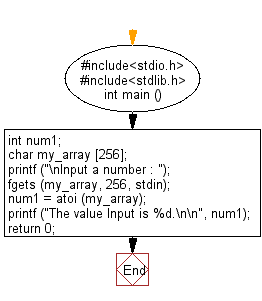﻿ C Program: Convert a string to an integer - w3resource

# C Exercises: Convert a string to an integer

## C Variable Type : Exercise-13 with Solution

Write a C program to convert a string to an integer.

Sample Solution:

C Code:

``````#include<stdio.h>      // Include the standard input/output header file.
#include<stdlib.h>     // Include the standard library header file.

int main ()          // Start of the main function.
{
int num1;          // Declare an integer variable 'num1'.
char my_array;   // Declare a character array 'my_array' with a maximum size of 256.

printf ("\nInput a number : ");   // Prompt the user to input a number.
fgets (my_array, 256, stdin);     // Read a line of input from the user and store it in 'my_array'.

num1 = atoi (my_array);   // Convert the string in 'my_array' to an integer and store it in 'num1'.

printf ("The value Input is %d.\n\n", num1);   // Print the value of 'num1'.

return 0;   // Return 0 to indicate successful execution of the program.
}   // End of the main function.
```
```

Sample Output:

```Input a number : 100
The value Input is 100.
```

Flowchart:C Programming Code Editor:

What is the difficulty level of this exercise?

Test your Programming skills with w3resource's quiz.

﻿